Chapter 2. Atoms, Molecules, and Ions

# 2.4 Chemical Formulas

### Learning Objectives

By the end of this section, you will be able to:

• Symbolize the composition of molecules using molecular formulas and empirical formulas
• Represent the bonding arrangement of atoms within molecules using structural formulas

A molecular formula is a representation of a molecule that uses chemical symbols to indicate the types of atoms followed by subscripts to show the number of atoms of each type in the molecule. (A subscript is used only when more than one atom of a given type is present.) Molecular formulas are also used as abbreviations for the names of compounds.

The structural formula for a compound gives the same information as its molecular formula (the types and numbers of atoms in the molecule) but also shows how the atoms are connected in the molecule. The structural formula for methane contains symbols for one C atom and four H atoms, indicating the number of atoms in the molecule (Figure 1). The lines represent bonds that hold the atoms together. (A chemical bond is an attraction between atoms or ions that holds them together in a molecule or a crystal.) We will discuss chemical bonds and see how to predict the arrangement of atoms in a molecule later. For now, simply know that the lines are an indication of how the atoms are connected in a molecule. A ball-and-stick model shows the geometric arrangement of the atoms with atomic sizes not to scale, and a space-filling model shows the relative sizes of the atoms.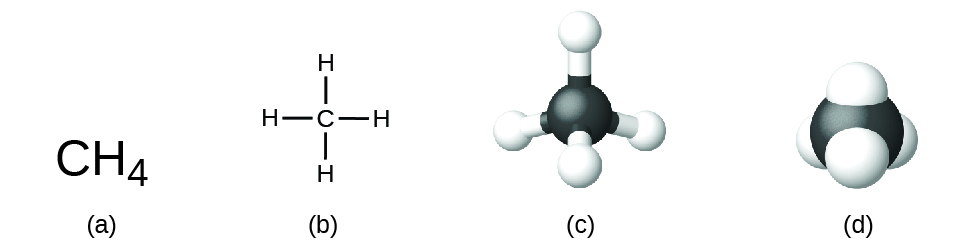Figure 1. A methane molecule can be represented as (a) a molecular formula, (b) a structural formula, (c) a ball-and-stick model, and (d) a space-filling model. Carbon and hydrogen atoms are represented by black and white spheres, respectively.

Although many elements consist of discrete, individual atoms, some exist as molecules made up of two or more atoms of the element chemically bonded together. For example, most samples of the elements hydrogen, oxygen, and nitrogen are composed of molecules that contain two atoms each (called diatomic molecules) and thus have the molecular formulas H2, O2, and N2, respectively. Other elements commonly found as diatomic molecules are fluorine (F2), chlorine (Cl2), bromine (Br2), and iodine (I2). The most common form of the element sulfur is composed of molecules that consist of eight atoms of sulfur; its molecular formula is S8 (Figure 2).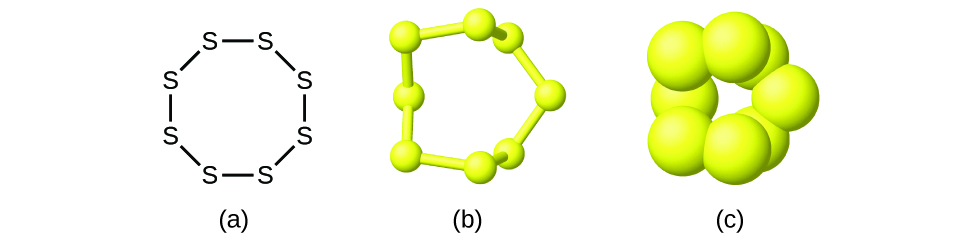Figure 2. A molecule of sulfur is composed of eight sulfur atoms and is therefore written as S8. It can be represented as (a) a structural formula, (b) a ball-and-stick model, and (c) a space-filling model. Sulfur atoms are represented by yellow spheres.

It is important to note that a subscript following a symbol and a number in front of a symbol do not represent the same thing; for example, H2 and 2H represent distinctly different species. H2 is a molecular formula; it represents a diatomic molecule of hydrogen, consisting of two atoms of the element that are chemically bonded together. The expression 2H, on the other hand, indicates two separate hydrogen atoms that are not combined as a unit. The expression 2H2 represents two molecules of diatomic hydrogen (Figure 3).

Compounds are formed when two or more elements chemically combine, resulting in the formation of bonds. For example, hydrogen and oxygen can react to form water, and sodium and chlorine can react to form table salt. We sometimes describe the composition of these compounds with an empirical formula, which indicates the types of atoms present and the simplest whole-number ratio of the number of atoms (or ions) in the compound. For example, titanium dioxide (used as pigment in white paint and in the thick, white, blocking type of sunscreen) has an empirical formula of TiO2. This identifies the elements titanium (Ti) and oxygen (O) as the constituents of titanium dioxide, and indicates the presence of twice as many atoms of the element oxygen as atoms of the element titanium (Figure 4).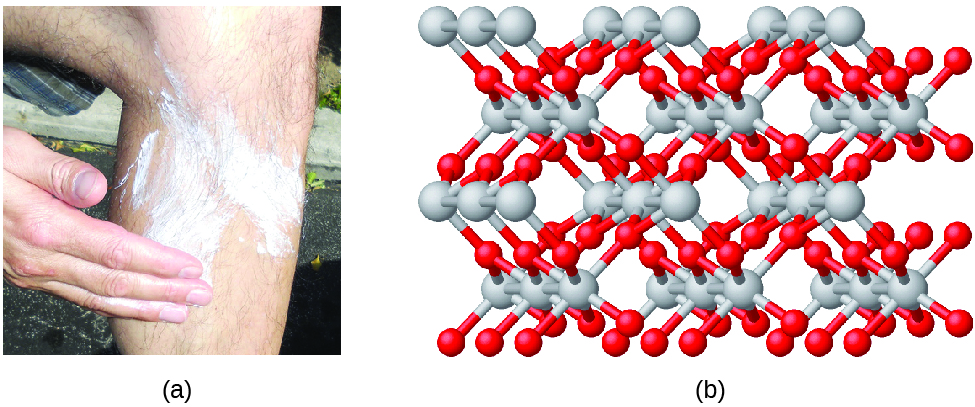Figure 4. (a) The white compound titanium dioxide provides effective protection from the sun. (b) A crystal of titanium dioxide, TiO2, contains titanium and oxygen in a ratio of 1 to 2. The titanium atoms are gray and the oxygen atoms are red. (credit a: modification of work by “osseous”/Flickr)

As discussed previously, we can describe a compound with a molecular formula, in which the subscripts indicate the actual numbers of atoms of each element in a molecule of the compound. In many cases, the molecular formula of a substance is derived from experimental determination of both its empirical formula and its molecular mass (the sum of atomic masses for all atoms composing the molecule). For example, it can be determined experimentally that benzene contains two elements, carbon (C) and hydrogen (H), and that for every carbon atom in benzene, there is one hydrogen atom. Thus, the empirical formula is CH. An experimental determination of the molecular mass reveals that a molecule of benzene contains six carbon atoms and six hydrogen atoms, so the molecular formula for benzene is C6H6 (Figure 5).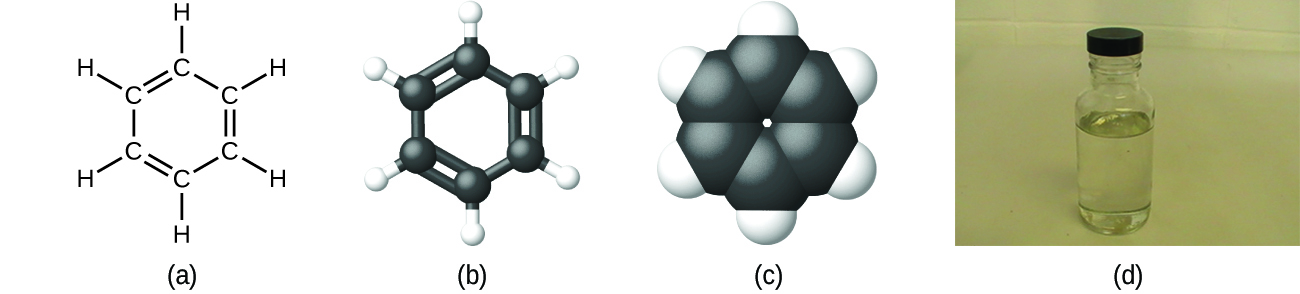Figure 5. Benzene, C6H6, is produced during oil refining and has many industrial uses. A benzene molecule can be represented as (a) a structural formula, (b) a ball-and-stick model, and (c) a space-filling model. (d) Benzene is a clear liquid. (credit d: modification of work by Sahar Atwa)

If we know a compound’s formula, we can easily determine the empirical formula. (This is somewhat of an academic exercise; the reverse chronology is generally followed in actual practice.) For example, the molecular formula for acetic acid, the component that gives vinegar its sharp taste, is C2H4O2. This formula indicates that a molecule of acetic acid (Figure 6) contains two carbon atoms, four hydrogen atoms, and two oxygen atoms. The ratio of atoms is 2:4:2. Dividing by the lowest common denominator (2) gives the simplest, whole-number ratio of atoms, 1:2:1, so the empirical formula is CH2O. Note that a molecular formula is always a whole-number multiple of an empirical formula.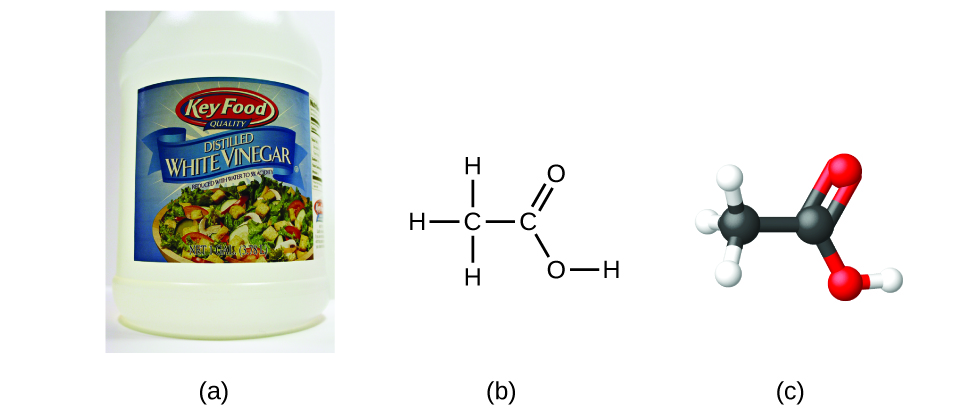Figure 6. (a) Vinegar contains acetic acid, C2H4O2, which has an empirical formula of CH2O. It can be represented as (b) a structural formula and (c) as a ball-and-stick model. (credit a: modification of work by “HomeSpot HQ”/Flickr)

### Example 1

Empirical and Molecular Formulas
Molecules of glucose (blood sugar) contain 6 carbon atoms, 12 hydrogen atoms, and 6 oxygen atoms. What are the molecular and empirical formulas of glucose?

Solution
The molecular formula is C6H12O6 because one molecule actually contains 6 C, 12 H, and 6 O atoms. The simplest whole-number ratio of C to H to O atoms in glucose is 1:2:1, so the empirical formula is CH2O.

A molecule of metaldehyde (a pesticide used for snails and slugs) contains 8 carbon atoms, 16 hydrogen atoms, and 4 oxygen atoms. What are the molecular and empirical formulas of metaldehyde?

Molecular formula, C8H16O4; empirical formula, C2H4OYou can explore molecule building using an online simulation.

### Lee Cronin

What is it that chemists do? According to Lee Cronin (Figure 7), chemists make very complicated molecules by “chopping up” small molecules and “reverse engineering” them. He wonders if we could “make a really cool universal chemistry set” by what he calls “app-ing” chemistry. Could we “app” chemistry?

In a 2012 TED talk, Lee describes one fascinating possibility: combining a collection of chemical “inks” with a 3D printer capable of fabricating a reaction apparatus (tiny test tubes, beakers, and the like) to fashion a “universal toolkit of chemistry.” This toolkit could be used to create custom-tailored drugs to fight a new superbug or to “print” medicine personally configured to your genetic makeup, environment, and health situation. Says Cronin, “What Apple did for music, I’d like to do for the discovery and distribution of prescription drugs.” View his full talk at the TED website.Figure 7. Chemist Lee Cronin has been named one of the UK’s 10 most inspirational scientists. The youngest chair at the University of Glasgow, Lee runs a large research group, collaborates with many scientists worldwide, has published over 250 papers in top scientific journals, and has given more than 150 invited talks. His research focuses on complex chemical systems and their potential to transform technology, but also branches into nanoscience, solar fuels, synthetic biology, and even artificial life and evolution. (credit: image courtesy of Lee Cronin)

It is important to be aware that it may be possible for the same atoms to be arranged in different ways: Compounds with the same molecular formula may have different atom-to-atom bonding and therefore different structures. For example, could there be another compound with the same formula as acetic acid, C2H4O2? And if so, what would be the structure of its molecules?

If you predict that another compound with the formula C2H4O2 could exist, then you demonstrated good chemical insight and are correct. Two C atoms, four H atoms, and two O atoms can also be arranged to form a methyl formate, which is used in manufacturing, as an insecticide, and for quick-drying finishes. Methyl formate molecules have one of the oxygen atoms between the two carbon atoms, differing from the arrangement in acetic acid molecules. Acetic acid and methyl formate are examples of isomers—compounds with the same chemical formula but different molecular structures (Figure 8). Note that this small difference in the arrangement of the atoms has a major effect on their respective chemical properties. You would certainly not want to use a solution of methyl formate as a substitute for a solution of acetic acid (vinegar) when you make salad dressing.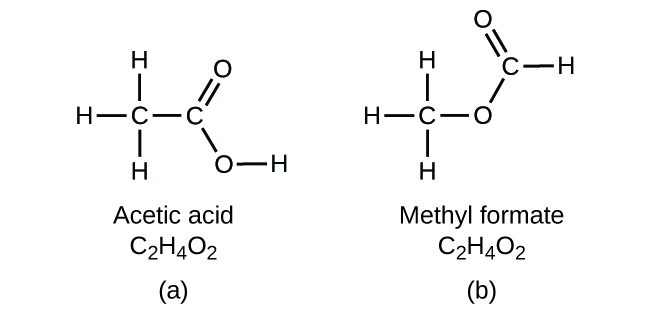Figure 8. Molecules of (a) acetic acid and methyl formate (b) are structural isomers; they have the same formula (C2H4O2) but different structures (and therefore different chemical properties).

Many types of isomers exist (Figure 9). Acetic acid and methyl formate are structural isomers, compounds in which the molecules differ in how the atoms are connected to each other. There are also various types of spatial isomers, in which the relative orientations of the atoms in space can be different. For example, the compound carvone (found in caraway seeds, spearmint, and mandarin orange peels) consists of two isomers that are mirror images of each other. S-(+)-carvone smells like caraway, and R-(−)-carvone smells like spearmint.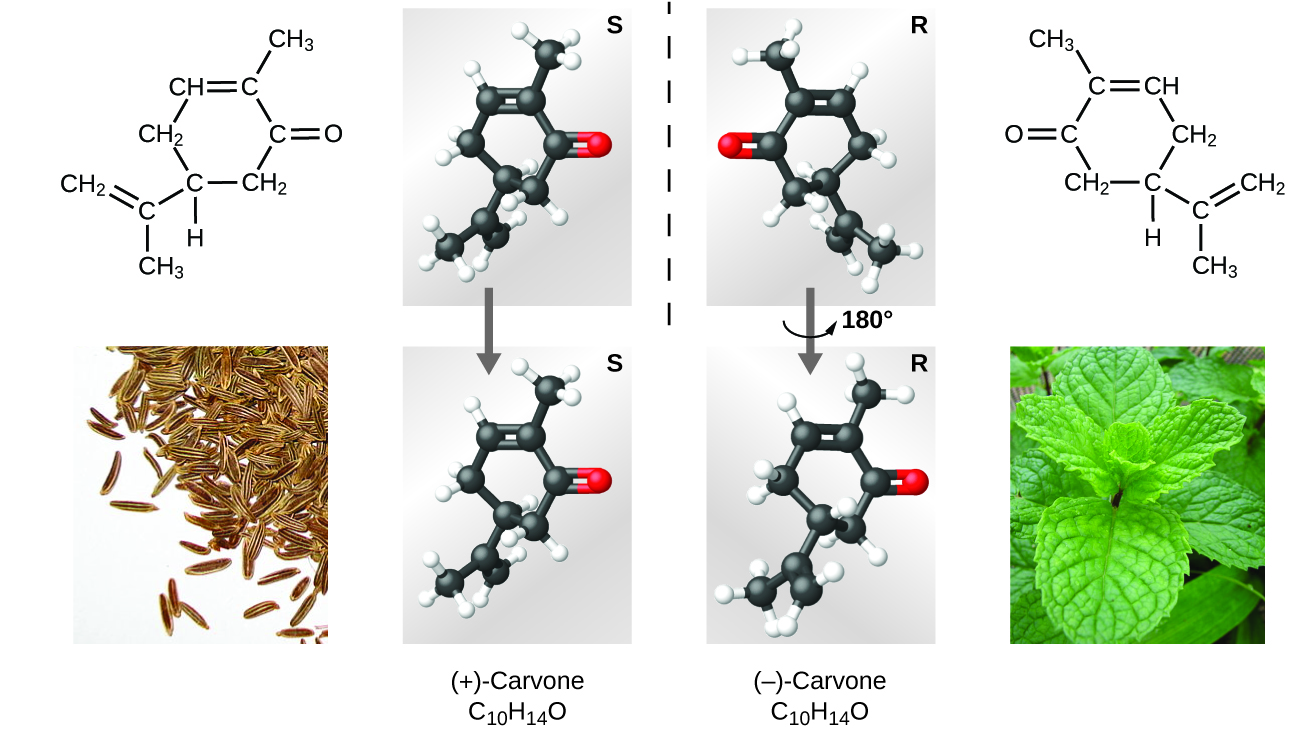Figure 9. Molecules of carvone are spatial isomers; they only differ in the relative orientations of the atoms in space. (credit bottom left: modification of work by “Miansari66”/Wikimedia Commons; credit bottom right: modification of work by Forest & Kim Starr)Select this link to view an explanation of isomers, spatial isomers, and why they have different smells (select the video titled “Mirror Molecule: Carvone”).

# Key Concepts and Summary

A molecular formula uses chemical symbols and subscripts to indicate the exact numbers of different atoms in a molecule or compound. An empirical formula gives the simplest, whole-number ratio of atoms in a compound. A structural formula indicates the bonding arrangement of the atoms in the molecule. Ball-and-stick and space-filling models show the geometric arrangement of atoms in a molecule. Isomers are compounds with the same molecular formula but different arrangements of atoms.

### Chemistry End of Chapter Exercises

1. Explain why the symbol for an atom of the element oxygen and the formula for a molecule of oxygen differ.
2. Explain why the symbol for the element sulfur and the formula for a molecule of sulfur differ.
3. Write the molecular and empirical formulas of the following compounds:

(a)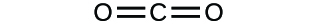(b)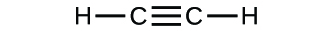(c)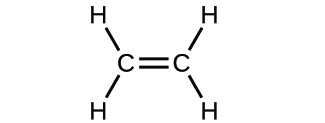(d)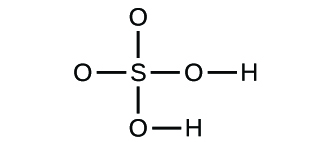4. Write the molecular and empirical formulas of the following compounds:

(a)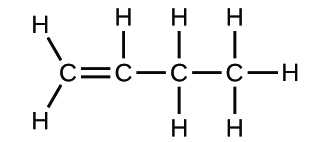(b)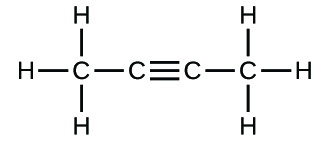(c)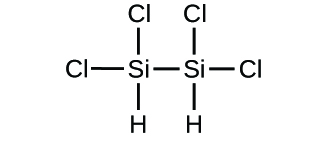(d)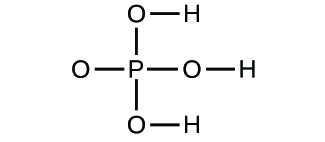5. Determine the empirical formulas for the following compounds:

(a) caffeine, C8H10N4O2

(b) fructose, C12H22O11

(c) hydrogen peroxide, H2O2

(d) glucose, C6H12O6

(e) ascorbic acid (vitamin C), C6H8O6

6. Determine the empirical formulas for the following compounds:

(a) acetic acid, C2H4O2

(b) citric acid, C6H8O7

(c) hydrazine, N2H4

(d) nicotine, C10H14N2

(e) butane, C4H10

7. Write the empirical formulas for the following compounds:

(a)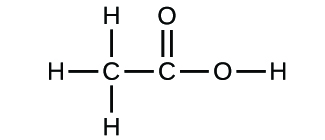(b)8. Open the Build a Molecule simulation and select the “Larger Molecules” tab. Select an appropriate atoms “Kit” to build a molecule with two carbon and six hydrogen atoms. Drag atoms into the space above the “Kit” to make a molecule. A name will appear when you have made an actual molecule that exists (even if it is not the one you want). You can use the scissors tool to separate atoms if you would like to change the connections. Click on “3D” to see the molecule, and look at both the space-filling and ball-and-stick possibilities.

(a) Draw the structural formula of this molecule and state its name.

(b) Can you arrange these atoms in any way to make a different compound?

9. Use the Build a Molecule simulation to repeat Chemistry End of Chapter Exercise 8, but build a molecule with two carbons, six hydrogens, and one oxygen.

(a) Draw the structural formula of this molecule and state its name.

(b) Can you arrange these atoms to make a different molecule? If so, draw its structural formula and state its name.

(c) How are the molecules drawn in (a) and (b) the same? How do they differ? What are they called (the type of relationship between these molecules, not their names).

10. Use the Build a Molecule simulation to repeat Chemistry End of Chapter Exercise 8, but build a molecule with three carbons, seven hydrogens, and one chlorine.

(a) Draw the structural formula of this molecule and state its name.

(b) Can you arrange these atoms to make a different molecule? If so, draw its structural formula and state its name.

(c) How are the molecules drawn in (a) and (b) the same? How do they differ? What are they called (the type of relationship between these molecules, not their names)?

## Glossary

empirical formula
formula showing the composition of a compound given as the simplest whole-number ratio of atoms
isomers
compounds with the same chemical formula but different structures
molecular formula
formula indicating the composition of a molecule of a compound and giving the actual number of atoms of each element in a molecule of the compound.
spatial isomers
compounds in which the relative orientations of the atoms in space differ
structural formula
shows the atoms in a molecule and how they are connected
structural isomer
one of two substances that have the same molecular formula but different physical and chemical properties because their atoms are bonded differently

### Solutions

Answers to Chemistry End of Chapter Exercises

1. The symbol for the element oxygen, O, represents both the element and one atom of oxygen. A molecule of oxygen, O2, contains two oxygen atoms; the subscript 2 in the formula must be used to distinguish the diatomic molecule from two single oxygen atoms.

3. (a) molecular CO2, empirical CO2; (b) molecular C2H2, empirical CH; (c) molecular C2H4, empirical CH2; (d) molecular H2SO4, empirical H2SO4

5. (a) C4H5N2O; (b) C12H22O11; (c) HO; (d) CH2O; (e) C3H4O3

7. (a) CH2O; (b) C2H4O

9. (a) ethanol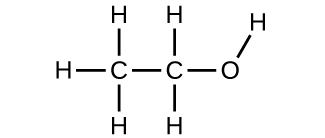(b) methoxymethane, more commonly known as dimethyl ether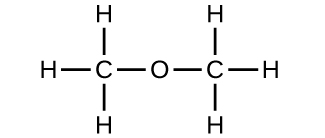(c) These molecules have the same chemical composition (types and number of atoms) but different chemical structures. They are structural isomers.

1. Lee Cronin, “Print Your Own Medicine,” Talk presented at TED Global 2012, Edinburgh, Scotland, June 2012.